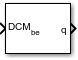Documentation

### This is machine translation

Mouseover text to see original. Click the button below to return to the English version of the page.

# Direction Cosine Matrix to Quaternions

Convert direction cosine matrix to quaternion vector

## Library

Utilities/Axes Transformations## Description

The Direction Cosine Matrix to Quaternions block transforms a 3-by-3 direction cosine matrix (DCM) into a four-element unit quaternion vector (q0, q1, q2, q3). The DCM performs the coordinate transformation of a vector in inertial axes to a vector in body axes.

The DCM is defined as a function of a unit quaternion vector by the following:

`$DCM=\left[\begin{array}{ccc}\left({q}_{0}^{2}+{q}_{1}^{2}-{q}_{2}^{2}-{q}_{3}^{2}\right)& 2\left({q}_{1}{q}_{2}+{q}_{0}{q}_{3}\right)& 2\left({q}_{1}{q}_{3}-{q}_{0}{q}_{2}\right)\\ 2\left({q}_{1}{q}_{2}-{q}_{0}{q}_{3}\right)& \left({q}_{0}^{2}-{q}_{1}^{2}+{q}_{2}^{2}-{q}_{3}^{2}\right)& 2\left({q}_{2}{q}_{3}+{q}_{0}{q}_{1}\right)\\ 2\left({q}_{1}{q}_{3}+{q}_{0}{q}_{2}\right)& 2\left({q}_{2}{q}_{3}-{q}_{0}{q}_{1}\right)& \left({q}_{0}^{2}-{q}_{1}^{2}-{q}_{2}^{2}+{q}_{3}^{2}\right)\end{array}\right]$`

Using this representation of the DCM, there are a number of calculations to arrive at the correct quaternion. The first of these is to calculate the trace of the DCM to determine which algorithms are used. If the trace is greater that zero, the quaternion can be automatically calculated. When the trace is less than or equal to zero, the major diagonal element of the DCM with the greatest value must be identified to determine the final algorithm used to calculate the quaternion. Once the major diagonal element is identified, the quaternion is calculated. For a detailed view of these algorithms, look under the mask of this block.

## Parameters

Action for invalid DCM

Block behavior when direction cosine matrix is invalid (not orthogonal).

• Warning — Displays warning and indicates that the direction cosine matrix is invalid.

• Error — Displays error and indicates that the direction cosine matrix is invalid.

• None — Does not display warning or error (default).

Tolerance for DCM validation

Tolerance of direction cosine matrix validity, specified as a scalar. Default is `eps(2)`. The block considers the direction cosine matrix valid if these conditions are true:

• The transpose of the direction cosine matrix times itself equals `1` within the specified tolerance (`transpose(n)*n == 1±tolerance`)

• The determinant of the direction cosine matrix equals `1` within the specified tolerance (`det(n) == 1±tolerance`).

## Inputs and Outputs

InputDimension TypeDescription

First

3-by-3 direction cosine matrix
OutputDimension TypeDescription

First

4-by-1 quaternion vector

#### Introduced before R2006a

##### SupportGet trial now